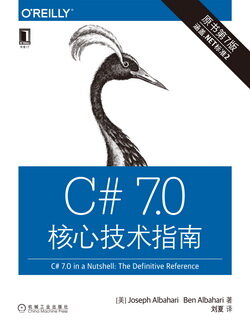# C# 7.0 核心技术指南 (23)：C#语言基础 2.4.2(数值转换)

#### 2.4.2.1 整数类型到整数类型的转换

int x = 12345;       // int is a 32-bit integerlong y = x;          // Implicit conversion to 64-bit integral typeshort z = (short)x;  // Explicit conversion to 16-bit integral type

#### 2.4.2.2 浮点类型到浮点类型的转换

double 能表示所有可能的 float 值，因此 float 能隐式转换为 double。反之则必须是显式转换。

#### 2.4.2.3 浮点类型到整数类型的转换

int i = 1;float f = i;

int i2 = (int)f;

int i1 = 100000001;float f = i1;          // Magnitude preserved, precision lostint i2 = (int)f;       // 100000000

#### 2.4.2.4 decimal 类型转换